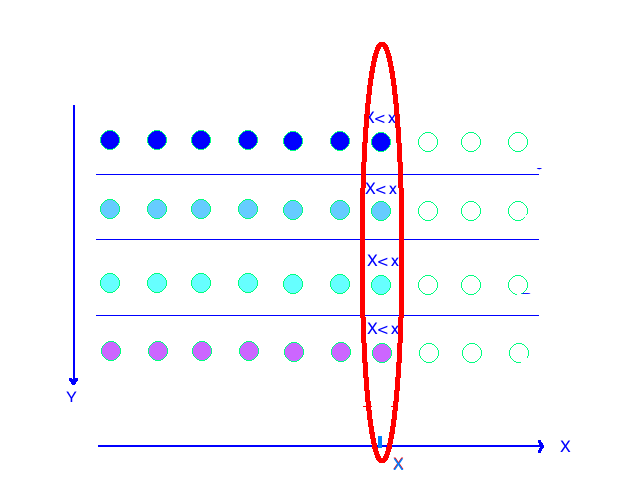Abstract: 本文承接上文，对于二维联合分布，如何求出二维变量中一个变量的一个分布，也就是标题所说的边缘分布；以及对独立随机变量的讨论。
Keywords: Marginal p.f.,Marginal p.d.f.,Independent

# 边缘分布

## 求边缘概率（分布）函数 Deriving a Marginal p.f. or a Marginal p.d.f.

### 离散分布边缘概率函数 Discrete

Head Tail $f_1(x)$
1 $\frac{1}{24}$ $\frac{3}{24}$ $\frac{1}{6}$
2 $\frac{2}{24}$ $\frac{2}{24}$ $\frac{1}{6}$
3 $\frac{3}{24}$ $\frac{1}{24}$ $\frac{1}{6}$
4 $\frac{1}{24}$ $\frac{3}{24}$ $\frac{1}{6}$
5 $\frac{1}{24}$ $\frac{3}{24}$ $\frac{1}{6}$
6 $\frac{2}{24}$ $\frac{2}{24}$ $\frac{1}{6}$
$f_2(y)$ $\frac{5}{12}$ $\frac{7}{12}$ 1

Definition Marginal c.d.f./p.f./p.d.f. Suppose that X and Y have a joint distribution.The c.d.f. of X derived by theorem 3.4.5. is called the marginal c.d.f. of X.Similarly,the p.f. or p.d.f. of X associated with the marginal c.d.f. of X is called the marginal p.f. or marginal p.d.f. of X

Theorem Let $X$ and $Y$ have a joint c.d.f. $F$.The c.d.f. $F_1$ of just the single random variable $X$ can be derived from the joint c.d.f. $F$ as $F_1(x)=lim_{y\to \infty}F(x,y)$.Similarly,the c.d.f. $F_2$ of $Y$ equals $F_2(y)=lim_{x\to \infty}F(x,y)$ ,for $0<y\leq \infty$

Theorem If $X$ and $Y$ have a discrete joint distribution for thich the joint p.f. is $f$,then the marginal p.f. $f_1$ of $X$ is
$$f_1(x)=\sum_{\text{All } y}f(x,y).$$
Similarly,the marginal p.f. $f_2$ of $Y$ is
$$f_2(y)=\sum_{\text{All } x}f(x,y).$$$f_1(x)$ 是在联合分布概率函数中，某一个变量不变（$x$） 而另一个变量取和的这种方式，来得到的边缘分布（x的一个一维分布概率函数）。如果从最初的关于概率的定义出发，我们也能得到类似的结论，二维随机变量对应的可以能是一个试验的二维结果，二维结果就对应了两个维度的可能性，而这个二维联合p.f.就是描述这个二维结果发生的概率的，当一个维度的可能性被消除（也就是二维变一维：$(x,y)\to x$）那么就应该把同一个 $x$ 下的所有不同的 $y$ 都加起来，因为在二维联合分布情况下 $(x_i,y_k)$ 和 $(x_i,y_l)$ 是不相关的（$k\neq l$）所以可以进行加法。

### 连续分布边缘概率密度函数 Continuous

Theorem If X and Y have a continuous joint distribution with joint p.d.f $f$ then the marginal p.d.f. f_1 of X is
$$f_1(x)=\int_{-\infty}^{\infty}f(x,y)dy \text{ for }-\infty<x<\infty$$
Similarly,the marginal p.d.f.f_2 of Y is
$$f_2(y)=\int_{-\infty}^{\infty}f(x,y)dx \text{ for }-\infty<y<\infty$$

$$F(x,y)=\int^{x}_{-\infty}\int^{y}_{-\infty}f(x,y)dydx$$

$$F(x)=\int^{x}_{-\infty}\int^{\infty}_{-\infty}f(x,y)dydx$$
F(x)和$f(x)$ 是导数与反导数的关系，所以，F(x) 求导可以得到：
$$f(x)=\frac{dF(x)}{dx}=\frac{d\int^{x}_{-\infty}\int^{\infty}_{-\infty}f(x,y)dydx}{dx}\\ \text{set :}\int^{x}_{-\infty}[\int^{\infty}_{-\infty}f(x,y)dy]dx=\int^{x}_{-\infty}g(x)dx\\ \text{so }f(x)=g(x)=\int^{\infty}_{-\infty}f(x,y)dy$$
Q.E.D

$$f(x,y)= \begin{cases} \frac{21}{4}x^2y&\text{for }x^2\leq y\leq 1\\ 0&\text{otherwise} \end{cases}$$

$$f_1(x)=\int^{\infty}_{-\infty}f(x,y)dy=\int^{1}_{x^2}\frac{21}{4}x^2ydy=(\frac{21}{8})x^2(1-x^4)$$

## 混合分布 Mixed

Theorem Let f be the joint p.f./p.d.f. of X and Y,with X discrete and Y continuous.Then the marginal p.f. of X is :
$$f_1(x)=Pr(X=x)=\int^{\infty}_{-\infty}f(x,y)dy \quad\text{for all }x$$
and the marginal p.d.f. of Y is:
$$f_2(y)=\sum_{x}f(x,y) \text{ for }-\infty<y<\infty$$

## 随机变量的独立 Independent Random Variables

$$Pr((x,y))=Pr(x)Pr(y)$$

Definition Independent Random Variables.It is said that two random variables $X$ and $Y$ are independent if,for every two sets $A$ and $B$ of real numbers such that ${x\in A}$ and ${Y\in B }$ are events,
$$Pr(X\in A \text{ and } Y \in B)=Pr(X\in A)Pr(Y\in B)$$

$$Pr(E\cap F)=Pr(E)Pr(F)$$

$$Pr(X\in{X<x})\\ Pr(Y\in{Y<y})\\ \text{so we have:}\\ Pr(X\leq x\text{ and }Y\leq y)=Pr(X\leq x)Pr(Y\leq y)$$

### 判定随机变量独立方法 1

Theorem Let the joint c.d.f. of $X$ and $Y$ be $F$ let the marginal c.d.f. of $X$ be $F_1$ and let the marginal c.d.f. of $Y$ be $F_2$ Then $X$ and $Y$ are independent if and only if ,for all real numbers $x$ and $y$ ,
$F(x,y)=F_1(x)F_2(y)$

### 判定随机变量独立方法 2

Theorem Suppose that $X$ and $Y$ are random varibales that have a joint p.f.,p.d.f. or p.f./p.d.f. $f$ ,Then $X$ and $Y$ will be independent if and only if $f$ can be represented in the following form for $-\infty<x<\infty$ and $-\infty<y<\infty$ :
$$f(x,y)=h_1(x)h_2(y)$$

• “if” part:
$$\text{ hold: } f(x,y)=h_1(x)h_2(y) \\ \text{marginal p.d.f. of }x\text{ : }f_1(x)=\int^{\infty}_{-\infty}h_1(x)h_2(y)dy=c_1h_1(x)$$
$c_1$ 是一个非负函数积分的结果，所以我们保证其是非负数，同时我们确定其有限，并且不是0，那么我们就能得到：
$$h_1(x)=\frac{f_1(x)}{c_1}$$
与此相似，我们用求和的方法求离散的边缘分布：
$$f_2(y)=\sum_{x}f(x,y)=\sum_{\text{All }x}h_1(x)h_2(y)=h_2(y)\sum_{x}\frac{1}{c_1}f_1(x)=\frac{1}{c}h_2(y)$$

$$f(x,y)=\frac{f_1(x)}{c_1} c_1 f_2(y)=f_1(x)f_2(y)$$

$$Pr(X\in A \text{ and } Y \in B)=\sum_{X \in A}\int_Bf(x,y)dy\\ =\int_B\sum_{X\in A}f_1(x)f_2(y)dy=\sum_{X\in A}f_1(x)\int_Bf_2(y)dy$$

• “only if” part:
we assume that X and Y are independent:
$$Pr(X\in A \text{ and } Y \in B)\\ =\sum_{X\in A}f_1(x)\int_Bf_2(y)dy\\ =\int_B\sum_{X\in A}f_1(x)f_2(y)dy$$
经过上面的分解，我们就能得到两个随机变量的概率
$$Pr(A)=h_1(x)=\sum_{X\in A}f_1(x)\\ Pr(B)=h_2(y)=\int_Bf_2(y)dy$$
这部分证明过程用到了上一篇混合随机变量的联合概率密度函数( $Pr(X\in A \text{ and } Y \in B)=\sum_{X\in A}f_1(x)\int_Bf_2(y)dy$ ) 然后通过微积分的基本过程得到了结论

Q.E.D

$$f(x,y)= \begin{cases} kx^2y^2&\text{for }x^2+y^2\leq 1\\ 0&\text{otherwise} \end{cases}$$
X,Y是否独立。

$f_1(0.9)f_2(0.9)=0$

### 判定随机变量独立方法 3

Theorem Let $X$ and $Y$ have a continuous joint distribution .Suppose that ${(x,y):f(x,y)>0}$ is a rectangular region $R$ (possibly unbounded) with sides (if any) parallel to the coordinate axes.Then X and Y are independent if and only if $f(x,y)=h_1(x)h_2(y)$ holds for all $(x,y)\in \Re^2$

### 判定随机变量独立方法 4

$$f(x,y)=e^xy\quad \text{ for } -\infty<x<0 \text{ and }0<y<1$$

$$Pr(X\in A \text{ and } Y \in B)=Pr(X\in A)Pr(Y\in B)$$

$$Pr(X\in {X<e^x} \text{ and } Y \in {Y<y})=e^xy$$

0%